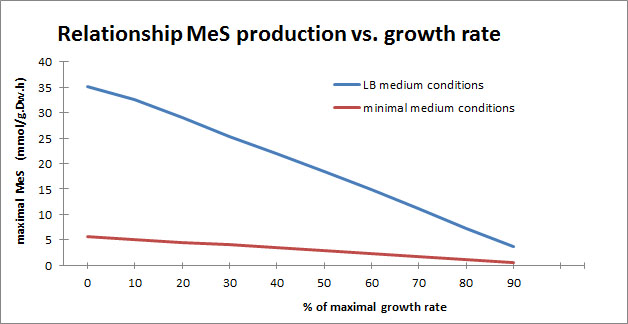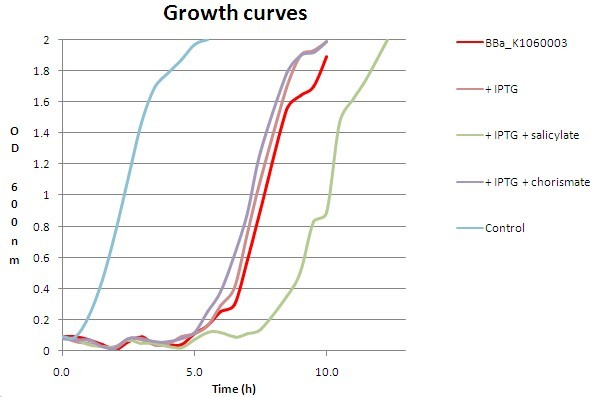# Team:KU Leuven/Project/Glucosemodel/MeS/Modelling-FBA

(diff) ← Older revision | Latest revision (diff) | Newer revision → (diff)

iGem

### Secret garden

Congratulations! You've found our secret garden! Follow the instructions below and win a great prize at the World jamboree!

• A video shows that two of our team members are having great fun at our favourite company. Do you know the name of the second member that appears in the video?
• For one of our models we had to do very extensive computations. To prevent our own computers from overheating and to keep the temperature in our iGEM room at a normal level, we used a supercomputer. Which centre maintains this supercomputer? (Dutch abbreviation)
• We organised a symposium with a debate, some seminars and 2 iGEM project presentations. An iGEM team came all the way from the Netherlands to present their project. What is the name of their city?

Now put all of these in this URL:https://2013.igem.org/Team:KU_Leuven/(firstname)(abbreviation)(city), (loose the brackets and put everything in lowercase) and follow the very last instruction to get your special jamboree prize!We needed to check whether the introduction of our MeS brick and the production of the components influences the overall BanAphid metabolism and/or growth rate. This modelling results will be checked against wetlab data, namely the growth curves we obtained while characterising our MeS biobricks. We also composed a Kinetic Parameter Model to estimate the average production rate of MeS. Approach and results can be found here.

A FBA calculates possibilities for the flow of metabolites through a metabolic network while maximising a set objective, in our case the growth rate of an organism or the production of a biotechnologically important metabolite. We ran the FBA for methyl salicylate using the COBRA Toolbox for MATLAB. COBRA stands for Constraint-Based Reconstruction and Analysis (COBRA) approach. It provides a biochemically and genetically consistent framework for the generation of hypotheses and the testing of functions of microbial cells. The Flux Balance Analysis (FBA) is probably the most used analysis within COBRA.

COBRA has been successfully applied to study the possible phenotypes that arise from a genome (Covert, Schilling et al. 2001; Orth et al. 2010). COBRA consists of two fundamental steps.
First, a GENRE (=GEnome-scale Network REconstruction) is formed, composed of the mathematical representation of all known metabolic reactions.
Second, the appropriate constraints are applied to form the corresponding GEMS (GEnome-scale Model in Silico).

Two fundamental types of constraints exist: balances and bounds (Price, Reed et al. 2004). Balance constraints are associated with conserved quantities such as energy, mass etc. Bounds limit numerical ranges of individual variables and parameters such as concentrations, fluxes or kinetic constants. At steady state, there is no accumulation or depletion of metabolites in a metabolic network, so the production rate of each metabolite in the network must equal its rate of consumption. This balance of fluxes can be represented mathematically as S . v = 0, where v is a vector of fluxes through the metabolic network and S is the stoichiometric matrix containing the stoichiometry of all reactions in the network.

Both bound and balance constraints limit the allowed functional states of reconstructed networks. Constraints can be very diverse in a biological system : physico-chemical constraints (reaction rates, enzyme turnover rates, diffusion rates etc.) , topo-biological (e.g. organisation of DNA in Escherichia coli by spatio-temporal patterns (Huang, Zhang et al. 2003)), environmental (nutrient availability, pH, temperature, osmolarity and the availability of electron acceptors and regulatory constraints).
In mathematical terms, the constraints define a system of linear equations which will be solved by linear programming in FBA. This will result in a range of allowable network states, described by a solution space which, in biology, represents the phenotypic potential of an organism. All allowable network states are contained in this solution space. (Covert and Palsson 2003; Price, Papin et al. 2003)
Thus, we can predict the growth rate potential of our BanAphids, defined by the constraints we impose, e.g. the growth medium, temperature, co-factor/precursor presence etc.

We used an E. coli model from 2007 (iAF1260 by Feist, AM. et al.) in all the following COBRA toolbox analyses.

As a first step we tried to predict the growth under default conditions for this model. This gave us the following results (after setting the biomass as objective function): for E. coli under default conditions a growth rate of 0.74 hr-1 is predicted. When performing the same calculations, but for LB medium conditions, a growth rate of 5.34 hr-1 is predicted. This shows that E. coli benefit from the LB medium conditions.

In a next step we wanted to add the reactions which are necessary for our model, but lacking in the iAF1260 model.
We added 'pchA', 'chor[c] -> ichor[c]' for the isochorismate synthesis reaction, 'pchB', 'ichor[c] -> sali[c] + pyr[c]' for the salicylate synthesis reaction and 'BSMT1', 'sali[c] -> methylsalicylate' for the methyl salicylate synthesis reaction.
We also added the exchange reaction for methylsalicylate ('Ex_methylsalicylate'). When we performed the growth calculation analysis for this modified model with the biomass set as objective function, we also observed a growth rate of 0.737 hr-1

Since our bacteria will be grown on LB medium, we changed the default medium settings towards those for LB medium. This means that we changed the relevant exchange reactions for the metabolites present in LB medium as seen in Tawornsamretkit et al.. The lower reaction bounds of the relevant reactions were set as following:
model = changeRxnBounds (model,'EX_glc(e)',0,'l')
model = changeRxnBounds (model,'EX_phe_L(e)',-0.1,'l')
model = changeRxnBounds (model,'EX_cys_L(e)',0,'l')
model = changeRxnBounds (model,'EX_ile_L(e)',-0.089,'l')
model = changeRxnBounds (model,'EX_ins(e)',-0.1,'l')
model = changeRxnBounds (model,'EX_hxan(e)',-0.1,'l')
model = changeRxnBounds (model,'EX_h2o(e)',-1000,'l')
model = changeRxnBounds (model,'EX_o2(e)',-1000,'l')
model = changeRxnBounds (model,'EX_co2(e)',-1000,'l')
model = changeRxnBounds (model,'EX_nh4(e)',-1000,'l')
model = changeRxnBounds (model,'EX_so4(e)',-1000,'l')
model = changeRxnBounds (model,'EX_ca2(e)',-1000,'l')
model = changeRxnBounds (model,'EX_h(e)',-1000,'l')
model = changeRxnBounds (model,'EX_k(e)',-1000,'l')
model = changeRxnBounds (model,'EX_mg2(e)',-1000,'l')
model = changeRxnBounds (model,'EX_na1(e)',-1000,'l')
model = changeRxnBounds (model,'EX_fe3(e)',-1000,'l')
model = changeRxnBounds (model,'EX_nac(e)',-1000,'l')
model = changeRxnBounds (model,'EX_thym(e)',-1000,'l')
model = changeRxnBounds (model,'EX_thr_L(e)',-0.288,'l')
model = changeRxnBounds (model,'EX_val_L(e)',-0.071 ,'l')
model = changeRxnBounds (model,'EX_pro_L(e)',-0.1,'l')
model = changeRxnBounds (model,'EX_his_L(e)',-1.642,'l')
model = changeRxnBounds (model,'EX_leu_L(e)',-0.1,'l')
model = changeRxnBounds (model,'EX_ura(e)',-1000,'l')
model = changeRxnBounds (model,'EX_tyr_L(e)',-0.035 ,'l')
model = changeRxnBounds (model,'EX_trp_L(e)',0,'l')
model = changeRxnBounds (model,'EX_met_L(e)',-0.1,'l')
model = changeRxnBounds (model,'EX_ser_L(e)',-1.722,'l')
model = changeRxnBounds (model,'EX_arg_L(e)',-1.17,'l')
model = changeRxnBounds (model,'EX_asp_L(e)',-0.041,'l')
model = changeRxnBounds (model,'EX_lys_L(e)',-0.1,'l')
model = changeRxnBounds (model,'EX_lys_L(e)',-0.1,'l')
model = changeRxnBounds (model,'EX_ala_L(e)',-0.369,'l')
model = changeRxnBounds (model,'EX_zn2(e)',-1000,'l')
model = changeRxnBounds (model,'EX_cd2(e)',-1000,'l')
model = changeRxnBounds (model,'EX_glyc(e)',-0.014,'l')
model = changeRxnBounds (model,'EX_gln_L(e)',-0.445,'l')
model = changeRxnBounds (model,'EX_glu_L(e)',0,'l')
model = changeRxnBounds (model,'EX_leu_L(e)',-0.235,'l')
model = changeRxnBounds (model,'EX_met_D(e)',-0.084,'l')
model = changeRxnBounds (model,'EX_met_L(e)',-0.084,'l')
model = changeRxnBounds (model,'EX_tre(e)',-0.6,'l')

When we performed the growth calculation with the biomass as objective function the flux to chorismate(a precursor of methyl salicylate) was 0.274 mol/hr in non-LB medium conditions and the growth of E. coli 0.737 hr-1. When we do the same but for the LB medium conditions we observe the flux to chorismate as 0.80 mol/hr and a growth rate of 5.34 hr-1. This suggests that LB-medium is beneficial for E. coli growth and improves the flux towards chorismate. Our aroG BioBrick (Part:BBa_K1060000) approach, aims however for a higher flux towards chorismate. This BioBrick contains mutations that can prevent the repression by Phenylalanine, that would occur otherwise and is in favour of chorismate production at the same time.

We were also interested to see how the maximal production of MeS is related to the maximal growth of E. coli under minimal conditions and in LB medium conditions. Therefore we set the objective function to MeS and set the lower bound for the biomass at different percentages of the maximal growth rate predicted with the biomass as objective function.As can be seen in the graph, there is a linear correlation between maximal flux towards MeS and maximal E. coli growth. This linear correlation is qualitatively similar both under minimal growth conditions as in LB medium conditions and shows that we are dealing with a trade off between bacterial growth and MeS production.
If a higher flux to MeS is preferred over a lower E. coli growth mass a value at the left side of the graph should be considered, whereas a value to the right would give a higher E. coli production rate and a lower flux towards MeS. This trade-off is reminiscent of our wet-lab growth curve results for the MeS brick.This figure shows how higher concentrations of added salicylate (0.1 mM) result in a longer lag phase. Higher levels of salicylate may lead to higher levels of S-adenosylmethionine (SAM) consumption, a co-substrate of the methyltransferase reaction producing methylsalicylate from salicylate. These higher consumption levels can in turn be associated with higher homocysteine levels, a side-product that stays behind when the methylgroup has been transferred to salicylate. Increased homocysteine levels are toxic for E. coli strains (Tuite et al. which may explain the ceiling we observe when adding salicylate, hoping for higher MeS fluxes.

FBA analysis predicted LB medium conditions to be beneficial for both E. coli growth and the flux towards chorismate, an important precursor for methylsalicylate. Moreover, a linear correlation between maximal predicted MeS flux and maximal growth rate exists, showing a trade off between the two. This linear correlation is present not only for LB medium conditions but also for minimal medium conditions and is qualitatively similar, showing a steeper correlation for LB medium conditions. These trade-offs are not only present in silico but we also found them in vivo in the choice between methyl salicylate production and growth rate. Finally, also our GC-MS analysis fits with this modelling, when salicylate was added to the medium, we not only observed a reduced growth rate but also a distinct MeS production peak.
These results not only show correlation between wetlab and modelling data but also suggest that cellular level modelling can result in colony wide effects (as observed in the delayed growth).

Covert, M. W. and B. O. Palsson (2003). "Constraints-based models: regulation of gene expression reduces the steady-state solution space." J Theor Biol 221(3): 309-325.
Covert, M. W., C. H. Schilling, I. Famili, J. S. Edwards, Goryanin, II, E. Selkov and B. O. Palsson (2001). "Metabolic modeling of microbial strains in silico." Trends Biochem Sci 26(3): 179-186.
Feist, AM et al. (2007) “A genome-scale metabolic reconstruction for Escherichia coli K-12 MG1655 that accounts for 1260 ORFs and thermodynamic information.” Mol. Syst. Biol. 3 121.
Huang, J., Q. Zhang and T. Schlick (2003). "Effect of DNA superhelicity and bound proteins on mechanistic aspects of the Hin-mediated and Fis-enhanced inversion." Biophys J 85(2): 804-817.
Iyarest Tawornsamretkit, Rattana Thanasomboon, Jittrawan Thaiprasit, Dujduan Waraho, Supapon Cheevadhanarak, Asawin Meechai, Analysis of Metabolic Network of Synthetic Escherichia coli Producing Linalool Using Constraint-based Modeling, Procedia Computer Science, Volume 11, 2012, Pages 24-35, ISSN 1877-0509Mahadevan, R. and C. H. Schilling (2003). "The effects of alternate optimal solutions in constraint-based genome-scale metabolic models." Metab Eng 5(4): 264-276.
Orth, J. D., I. Thiele, and B.O. Palsson (2010). "What is Flux Balance Analysis?" Nature Biotechnology (28): 245-248.
Papin, J. A., N. D. Price, J. S. Edwards and B. B. Palsson (2002). "The genome-scale metabolic extreme pathway structure in Haemophilus influenzae shows significant network redundancy." J Theor Biol 215(1): 67-82.
Price, N. D., J. A. Papin, C. H. Schilling and B. O. Palsson (2003). "Genome-scale microbial in silico models: the constraints-based approach." Trends Biotechnol 21(4): 162-169.
Price, N. D., J. L. Reed and B. O. Palsson (2004). "Genome-scale models of microbial cells: evaluating the consequences of constraints." Nat Rev Microbiol 2(11): 886-897.
Schilling, C. H., M. W. Covert, I. Famili, G. M. Church, J. S. Edwards and B. O. Palsson (2002). "Genome-scale metabolic model of Helicobacter pylori 26695." J Bacteriol 184(16): 4582-4593.
Tuite, N. L.,Fraser, K. R.,O'Byrne, C. P.(2005)."Homocysteine toxicity in Escherichia coli is caused by a perturbation of branched-chain amino acid biosynthesis" Journal of bacteriology 187(13):4362-4371.